Electron. J. Differential Equations, Vol. 2017 (2017), No. 29, pp. 1-19.

### A characterisation of infinity-harmonic and p-harmonic maps via affine variations in L-infinity Nikos Katzourakis

Abstract:
Let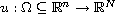be a smooth map and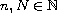. The-Laplacian is the PDE system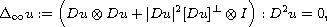where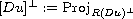. This system constitutes the fundamental equation of vectorial calculus of variations in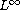, associated with the model functional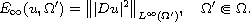We show that generalised solutions to the system can be characterised in terms of the functional via a set of designated affine variations. For the scalar case N=1, we utilize the theory of viscosity solutions by Crandall-Ishii-Lions. For the vectorial case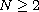, we utilize the recently proposed by the author theory of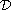-solutions. Moreover, we extend the result described above to the p-Laplacian,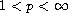.

Submitted August 10, 2016. Published January 26, 2017.
Math Subject Classifications: 35D99, 35D40, 35J47, 35J47, 35J92, 35J70, 35J99.
Key Words: Infinity-Laplacian; p-Laplacian; generalised solutions; viscosity solutions; calculus of variations in L-infinity; Young measures; fully nonlinear systems.

Show me the PDF file (338 KB), TEX file for this article.Nikos Katzourakis Department of Mathematics and Statistics University of Reading, Whiteknights, PO Box 220 Reading RG6 6AX, Berkshire, UK email: n.katzourakis@reading.ac.uk